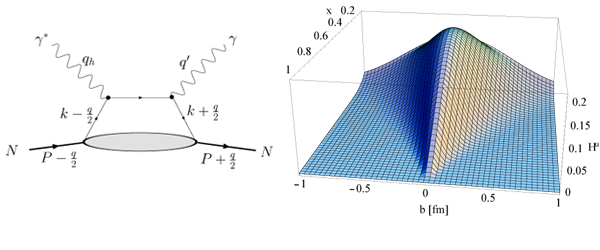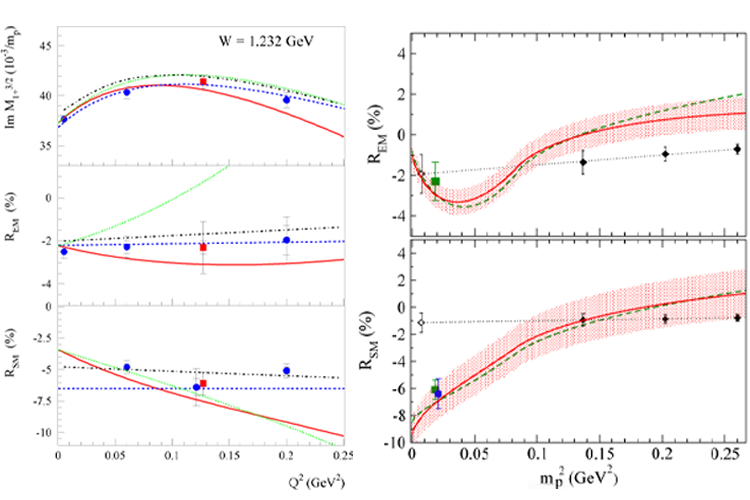# Forschungs-Highlights

## Nucleon Electromagnetic Form Factors

Electromagnetic form factors (FFs) of the nucleon are the standard source of information on the nucleon structure and as such have been studied extensively. The most recent generation of electron accelerators, which combine high current with high polarization electron beams, at MIT-Bates, the Mainz Microtron (MAMI), and the Continuous Electron Beam Accelerator Facility (CEBAF) of the Jefferson Lab (JLab), have made it possible to investigate the internal structure of the nucleon with unprecedented precision. In particular, the new generation of polarization experiments that make use of the target- and recoil-polarization techniques have allowed to chart the proton and neutron electromagnetic (e.m.) FFs very precisely.

Given the large amount of precise data on FFs it is of interest to exhibit directly the spatial information which results from these data. When viewing the nucleon from a light front moving towards the nucleon, a model independent 2-dimensional mapping of the quark charge density in the nucleon can be achieved in the transverse plane (perpendicular to the direction of motion). Using only the empirical information on the nucleon e.m. FFs, these transverse charge densities have been extracted recently for an unpolarized nucleon, as well as for a transversely polarized nucleon, see Figure 1. In the latter case, one notices that polarizing the proton along the x-axis leads to an induced electric dipole field which corresponds with an electric dipole moment along the y-axis equal to the value of the anomalous magnetic moment, κp = 1.79 (in units e/2 MN). The corresponding neutron's transverse charge density gets displaced significantly due to the large (negative) value of the neutron anomalous magnetic moment, κn = -1.91, which yields an induced electric dipole moment along the negative y-axis.Figure 1: Quark transverse charge densities in the proton (left panels) and neutron (right panels), extracted from the empirical parameterizations of proton and neutron form factor data. The upper panel shows the density in the transverse plane for an unpolarized nucleon, whereas the lower panels show the density for a nucleon polarized along the x-axis. The light (dark) regions correspond with largest (smallest) values of the density. The lower panel compares the density along the y-axis for an unpolarized nucleon (dashed curve), and for a nucleon polarized along the x-axis (solid curve).

References:

C.F. Perdrisat, V. Punjabi and M. Vanderhaeghen,

G.A. Miller,

C.E. Carlson and M. Vanderhaeghen, Phys. Rev. Lett. 100, 032004 (2008); arXiv:0807.4537 [hep-ph] .

## Generalized Parton Distributions

The nucleon e.m. FFs access the quark-gluon structure of the nucleon by measuring the matrix element of a well-defined quark-gluon operator (in this case the vector operator ‾q γμ q) over the hadronic state. One is however not limited in nature to probes such as photons (or W, Z bosons for the axial transition). The phenomenon of asymptotic freedom of QCD, meaning that at short distances the interactions between quarks and gluons become weak, provides us with more sophisticated QCD operators to explore the structure of hadrons. Such operators can be accessed by selecting a small size configuration of quarks and gluons, provided by a hard reaction, such as deep inelastic scattering (DIS), or hard exclusive reactions such as deeply virtual Compton scattering (DVCS), γ*(qh) + N(p) → γ (q') + N(p'), where the virtual photon momentum qh is the hard scale, see left panel of Figure 2. The common important feature of such hard reactions is the possibility to separate clearly the perturbative and nonperturbative stages of the interactions: this is the so-called factorization property. The non-perturbative stage of such hard exclusive electroproduction processes is described by universal objects, so-called generalized parton distributions (GPDs).

These GPDs have the physical meaning of measuring the probability to find a quark which carries longitudinal momentum fraction x at a transverse position b (relative to the transverse center-of-momentum) in a nucleon. The right panel of Figure 3 shows the GPD Hu, for an u-quark, within a 3-parameter modified Regge GPD parameterization.Figure 2: Left panel: handbag diagram for the nucleon DVCS process. Provided the virtuality of the initial photon (with momentum qh) is sufficiently large, the QCD factorization theorem allows to express the total amplitude as the convolution of a Compton process at the quark level and a non-perturbative amplitude parameterized in terms of GPDs (lower blob). Right panel : the GPD Hu for a valence up-quark in the proton as function of the quark momentum fraction x and the quark position b in the transverse plane (perpendicular to the average direction of the fast moving nucleons). The calculation is based on the 3-parameter modified Regge parametrization for the GPDs.

References :

K. Goeke, M.V. Polyakov and M. Vanderhaeghen, Prog. Part. Nucl. Phys. 47, 401 (2001).

M. Diehl, Phys. Rept. 388, 41 (2003).

A.V. Belitsky and A.V. Radyushkin, Phys. Rept. 418, 1 (2005).

M. Guidal, M.V. Polyakov, A.V. Radyushkin and M. Vanderhaeghen, Phys. Rev. D 72, 054013 (2005).

## Nucleon Excitation Spectrum

The understanding of the nucleon excitation spectrum is tightly linked to its structure. The first baryon excited state, the Δ(1232) resonance has been charted in particular detail in recent years. At low Q2, the measurement of γ N Δ FFs have allowed to unambiguously map out the small E2 and C2 amplitudes revealing d-wave components in the nucleon and/or Δ wave functions. In parallel, recent years have also seen the development of a chiral effective field theory (χEFT) with two distinct light mass scales : the pion mass and the Δ - N mass difference.

In Figure 3, a next-to-leading order (NLO) χEFT results for the Q2 dependence of the γ N Δ resonant multipoles, at the resonance position, characterized by magnetic dipole (M1), electric quadrupole (E2) and Coulomb quadrupole (C2) transitions. The red solid curve is with and the green dotted curve without the chiral-loop corrections. We observe from the figure that the chiral loops play a crucial role inthe low momentum-transfer dependence of the E2/M1 (REM) ratio. The effect of the pion cloud is most pronounced in the E2 γ N Δ transition.

The chiral EFT was found to be useful in a dual way: both to describe observables and as a tool to extrapolate lattice results. The latter is demonstrated on the right panel of Figure 3 which shows the mπ-dependence of the ratios REM and RSM within the χEFT framework in comparison with the lattice QCD calculations. It is seen in particular that the opening of the decay channel due to Δ → π N decay, at mπ = MΔ - MN, leads to strong non-analytic behavior in quark mass. For lattice studies, the Δ(1232) resonance, as a purely elastic resonance, is an ideal test case to study the issues, due to opening of decay channels, which are relevant for the whole baryonic spectrum.Figure 3: Left panel : resonant multipoles of pion electroproduction as function Q2 at the Δ-resonance position. Dotted curves : Δ-contribution alone. Solid curves : results of the NLO calculation of Ref. . Also shown are results of the SAID analysis (FA04K) (dashed-dotted curves), and the MAID 2003 analysis (dashed curves). The data points are from BATES (red solid square) and MAMI (blue solid circles). Right panel : pion mass dependence of E2/M1 (REM) (upper panel) and C2/M1 (RSM) (lower panel), at Q2=0.1 GeV2. Data points from MAMI (blue circle) and BATES (green squares). The three filled black diamonds at larger mπ are lattice calculations, whereas the open diamond near mπ ≂ 0 represents their extrapolation assuming linear dependence in mπ2. The red solid curves are a NLO effective field theory calculation, and the error bands are an estimate of the theoretical uncertainty.

References :

 V. Pascalutsa, M. Vanderhaeghen and S.N. Yang, Phys. Rept. 437, 125 (2007) .

 V. Pascalutsa and D.R. Phillips, Phys. Rev. C 67, 055202 (2003).

 V. Pascalutsa and M. Vanderhaeghen,Phys. Rev. Lett. 95, 232001 (2005); Phys. Rev. D 73, 034003 (2006).

 D. Drechsel, O. Hanstein, S.S. Kamalov and L. Tiator, Nucl. Phys. A 645, 145 (1999).